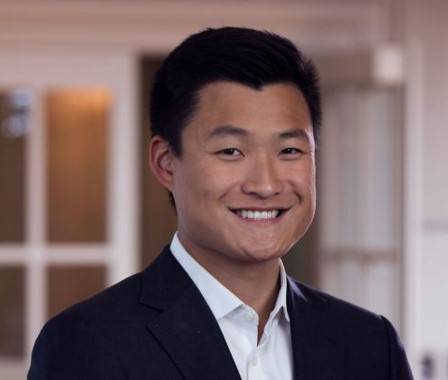Search
•Matt Ting

# Rule of 72 (How to Quickly Calculate IRR)

The Rule of 72 is a clever mathematical formula that can be used to determine an investment's compound growth rate. The Rule of 72 approximates the annual return of an investment, making it extremely useful for Paper LBOs. The Rule of 72 is just a mathematical formula and can be applied to anything that grows, such as the economy, a company's EBITDA, population, number of Instagram followers, etc.

The Rule of 72 estimates the number of years required to double the value of an investment at a fixed compound growth rate.

To use the Rule of 72, we divide 72 by the number of years that an investment is held for. Note that the Rule of 72 only works if the investment doubles in value over the course of the period.

• Question: What is the IRR of an investment that doubles in 5 years?

• Answer: We simply take 72 and divide by 5, as the investment doubles over 5 years. The answer is 14% IRR. If you were to calculate this in Excel, you would realize the actual IRR is 15%.

We can also use the Rule of 72 to determine the number of years that are required for a number to double at a given growth rate.

• Question: We forecast our portfolio company's EBITDA to grow at 8% annually. How long will it take for the company's EBITDA to double?

• Answer: We divide 72 by 8%, which is 9. Therefore, it would take 9 years of 8% annual growth for EBITDA to double. Yes, it's that simple.

We can also use the Rule of 114 for 3x and the Rule of 144 for 4x (which is just the Rule of 72 twice). Said more explicitly, we can calculate the rate that something needs to grow at to triple by using the Rule of 114.

## Rule of 72 MoM and IRR Chart• Question: Our investment has increased from \$250mm to \$750mm over the course of 10 years. What is the approximate IRR of the investment?

• Answer: We can quickly calculate that the value of the investment tripled (\$750 / \$250 = 3). We then use the Rule of 114, dividing 114 by 10 years. As a result, we get 11% (actual IRR is 12%).

Learn how to do a full LBO and prepare for case study interviews in our Private Equity Recruiting Course.